# PRODUCT Excel FunctionArticle byExcelMojo Team## What Is PRODUCT Function In Excel?

The PRODUCT function in Excel is an inbuilt Math & Trig function that performs the multiplication operation. It determines the product of the numbers supplied as arguments. Users will find the PRODUCT Excel function useful when they multiply multiple cell values in a wide cell range, including empty cells and text data.

For example, the below table contains sets of three numbers. Column D shows the requirements to determine the product of the numbers in each set. And we need to display the products in column E. Then, we can apply the PRODUCT() in the target cells and achieve the required output.

The column E formulas containing the PRODUCT Excel function return the values obtained by multiplying the required numbers in each row. For instance, the PRODUCT() in cell E2 multiplies the first two numbers in the second row, 10 and 20, to return the result as 200.

###### Key Takeaways
• The PRODUCT Excel function multiplies the supplied argument values to return their product.
• The function is useful when multiplying cell ranges containing empty cells and text values.
• Remember, the PRODUCT function can multiply positive and negative integers, decimal values, and fractional numbers. And we can also supply them directly as the values or as arrays, ranges, or cell references to the number values.
• The PRODUCT excel function takes one mandatory argument, number1, and 254 optional arguments, number2, …, as input.

### PRODUCT() Excel Formula

The PRODUCT Excel function syntax is:

where,

• number1: The first number or the cell range we require to multiply.
• number2, …: The other numbers or cell range we require to multiply.

While the first argument in the PRODUCT Excel function syntax is mandatory, the remaining arguments are optional.

Further, below are the critical aspects of the PRODUCT() that we must know to avoid potential errors.

• We can enter a maximum of 255 PRODUCT Excel function arguments.
• The PRODUCT() ignores the text, logical values, and empty cells. It only counts and multiplies the numbers in the given cell references, cell ranges, and arrays.
• If all the supplied PRODUCT Excel function arguments are non-numeric values, the function output will be 0.

### How To Use PRODUCT Excel Function?

The steps to apply the PRODUCT Excel function are as follows:

1. First, confirm the source table contains all the numbers you need to multiply.
2. Then, select the required target cell and enter the PRODUCT Excel function.
3. Finally, press Enter to execute the function.

The below example will explain the PRODUCT Excel function definition and the above steps in detail.

The table below shows multiple sets of six input values.

And suppose the requirement is to find the product of the values in each row and display the results in column G. Then, here is how we can apply the PRODUCT() in the target cells and achieve the required data.

1. Select the target cell G2, enter the below formula, and press Enter.

=PRODUCT(A2:F2)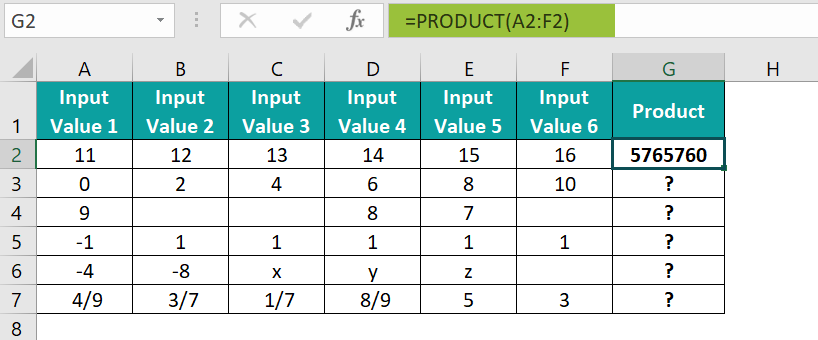On the other hand, directly supplying the numbers or cell references to individual numbers as the function arguments will also result in the same product value.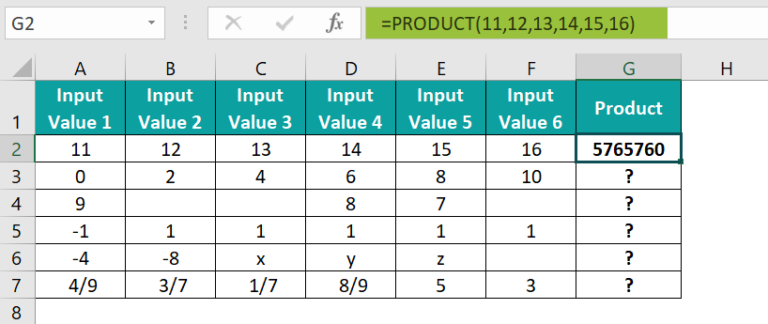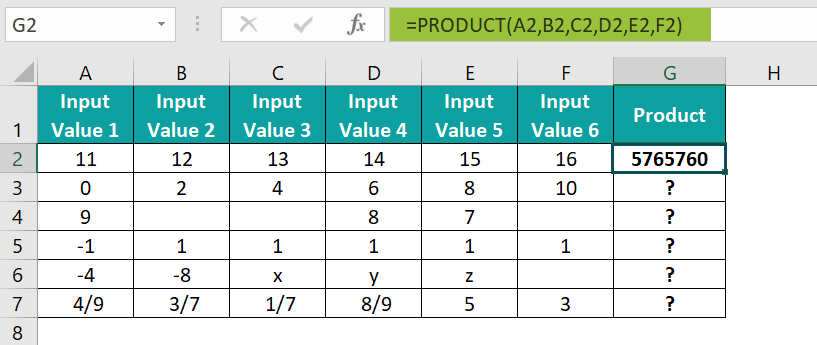Alternatively, we can apply the PRODUCT() from the Formulas tab. And for that, we must select the target cell G2 and click FormulasMath & TrigPRODUCT to open the Function Arguments window.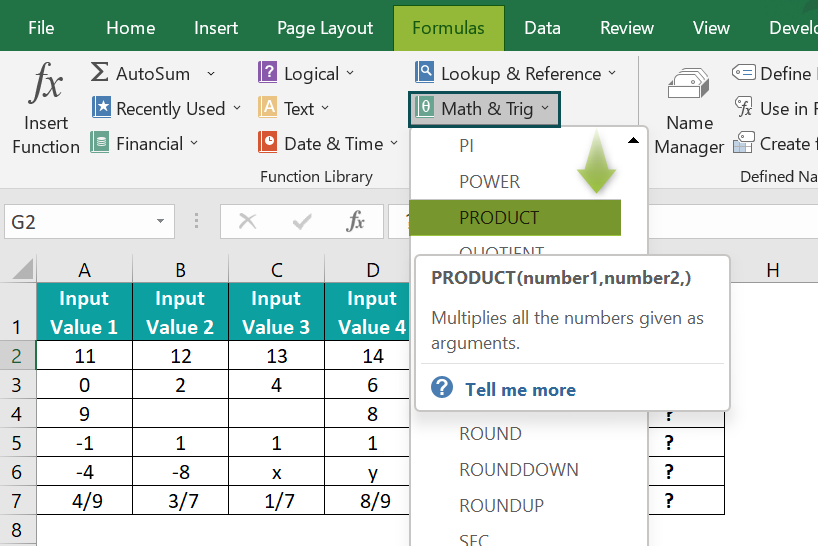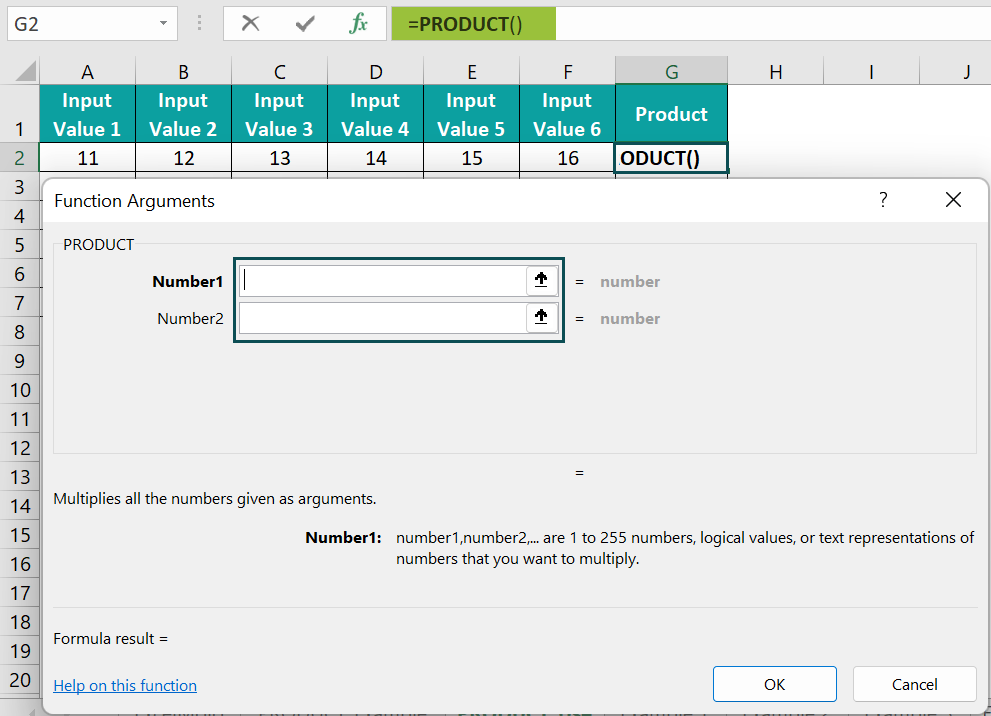Next, enter the PRODUCT() argument values in the Function Arguments window. Though we will see only two fields, the third field will appear once we click the second field. And we can continue entering as many numbers as we need to multiply, with the limit being 255 arguments.

In this case, we have to enter six argument values.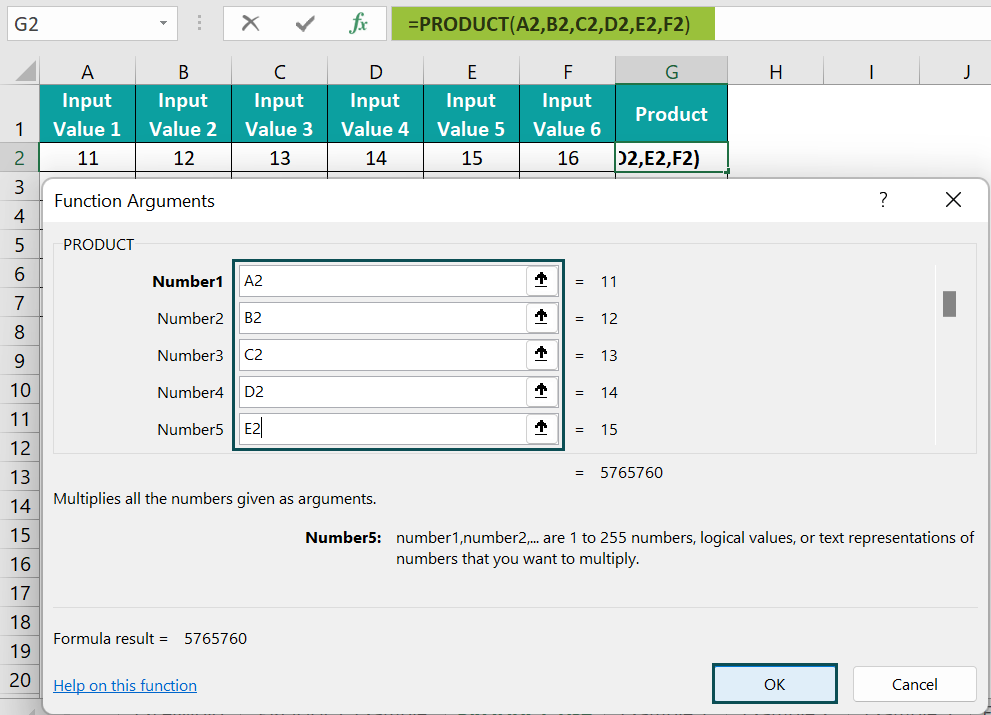And once we click OK in the Function Arguments window, the function will get executed.

We can also enter the argument value as a cell range in the first field and click OK to get the result.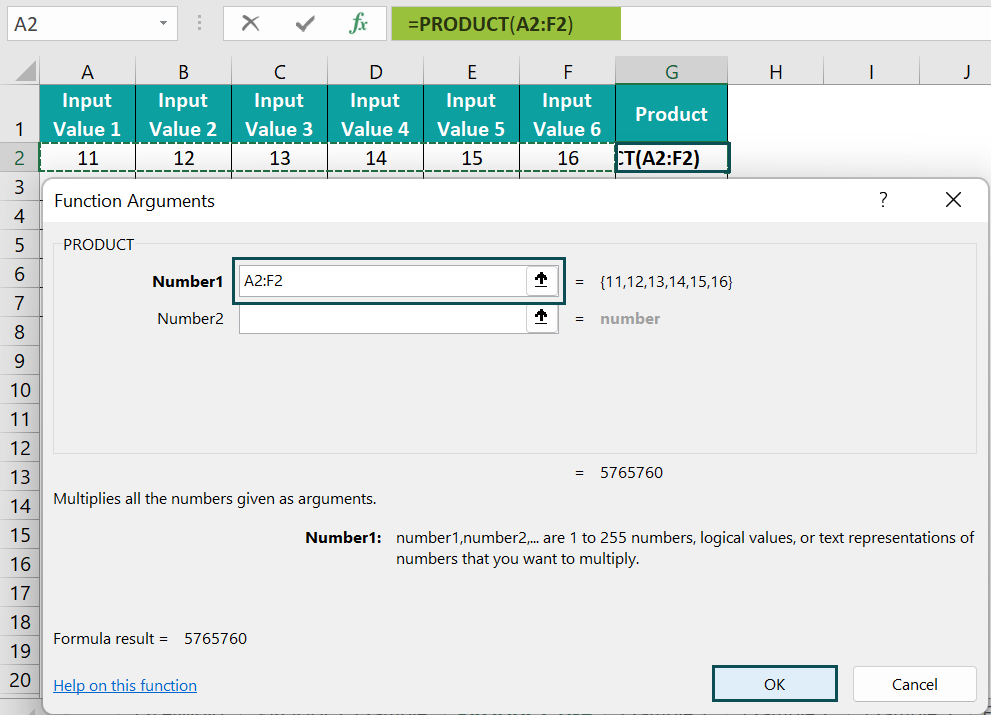2. Using the fill handle, copy the formula in cell range G3:G7.

=PRODUCT(A7:F7)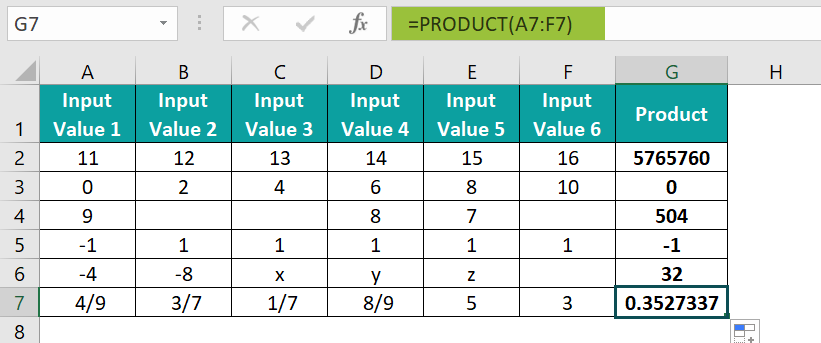The above formulas containing the PRODUCT Excel function return the product of the six values in each row, whether the numbers are positive, negative, or fractions.

The cell G3 output is 0, as one of the six values is 0 (cell A3). On the other hand, the cell G4 formula ignores the empty cells in the input range and returns the product of the values 9, 8, and 7, 504.

And in row 5, as one of the six values is a negative number (cell A5), the resulting product in cell G5 is a negative value. And the cell G6 formula ignores the text values and the empty cell in the input cell range to return the product of only the number values -4 and -8, 32.

Finally, the cell G7 formula returns the product of the given fractions and numbers, 0.3527337.

### Examples

The below examples explain the best ways to apply the PRODUCT Excel function.

#### Example #1

The following PRODUCT Excel function example shows we can use the PRODUCT() to multiply numbers in different cell ranges.

The image below shows two tables. The first table contains the 5-week results for three tests.

And suppose the requirements are to get the product values, as mentioned in the second table, to display the results for review in the cell range B10:B11. Then, we can apply the PRODUCT Excel function in the target cells and get the required figures.

• Step 1: Select the target cell B10, enter the below formula, and press Enter.

=PRODUCT(B2:B6,D2:D6)

The PRODUCT Excel function multiplies all the numbers in the supplied cell ranges, B2:B6 and D2:D6, and returns the value 0.0833976 as the required product.

• Step 2: Select the target cell B11, enter the below formula, and press Enter.

=PRODUCT(B4:B6,C4:C6,D4:D6)

In this case, as we need to multiply all test results from Week 3, the input cell ranges are B4:B6, C4:C6, and D4:D6. And thus, the resulting product of the nine values is 3.9405366.

The following formula will also result in the same product value.

=PRODUCT(B4:D6)

#### Example #2

The PRODUCT Excel function example below explains how to apply the PRODUCT() with other Excel functions such as the SUM and AVERAGE Excel function.

The following table contains the six months’ inventory data of apples.

And suppose the requirement is to determine the total apple quantity and the average apple price per carton based on the above table. And then, calculate the total apple price to display all the above data in cells B10:B11 and B13.

Then, we can apply the PRODUCT() with the AVERAGE and SUM Excel functions in the target cells and achieve the required data.

• Step 1: Select cell B10, enter the below formula, and press Enter.

=SUM(B2:B7)

• Step 2: Select cell B11, enter the below formula, and press Enter.

=AVERAGE(C2:C7)

• Step 3: Select cell B13, enter the following PRODUCT Excel function, and press Enter.

=PRODUCT(SUM(B2:B7),AVERAGE(C2:C7))

First, the SUM() returns the total quantity of Apples produced from January to June, 48. Then, the AVERAGE() returns the average price per carton based on the given data in the cell range, C2:C7, 24. And finally, the PRODUCT() multiplies the SUM(), and AVERAGE() outputs to return the required product value, \$1152.

#### Example #3

Here is how we can use the PRODUCT() with the VLOOKUP Excel function.

The following image shows three tables. The first table shows a list of models and their respective quantities produced monthly. And the second table contains the factors by which each model’s quantity produced monthly should increase.

Suppose we must multiply the quantity produced by the production increase factor for each model and display the result in column H of the third table. Then, applying the PRODUCT Excel function with the VLOOKUP() in the target cells we can get the required information.

• Step 1: Select cell H2, enter the below formula, and press Enter.

=PRODUCT(VLOOKUP(G2,A:B,2,0),VLOOKUP(G2,D:E,2,0))

• Step 2: Using the fill handle, copy the formula in the range H3:H11.

Let us consider the cell H11 formula to see how the formula works. The first VLOOKUP() looks up the quantity produced value in the first table for the model specified in cell G2 to return the value 100. And the second VLOOKUP() looks up the production increase factor in the second table for the model specified in cell G2 to return the value 4.

And finally, the PRODUCT() multiplies the two VLOOKUP() outputs to return the expected net monthly production of the model VHC7525 as 400.

### Important Things To Note

• The maximum number of arguments can supply as input to the PRODUCT Excel function is 255.
• The PRODUCT() does not consider the text, logical values, and blank cells. But it counts cells containing the zero value.
• If all the entered argument values are non-numeric, the PRODUCT() output will be 0.
• If any of the supplied argument values results in an error value, the PRODUCT() throws an error.
• The PRODUCT function yields excellent results when used with other inbuilt Excel functions, such as SUM, AVERAGE, and VLOOKUP.

1. Where is the PRODUCT function in Excel?

The PRODUCT function in Excel is in the Formulas tab. We can select FormulasMath & TrigPRODUCT to apply it in the required cell.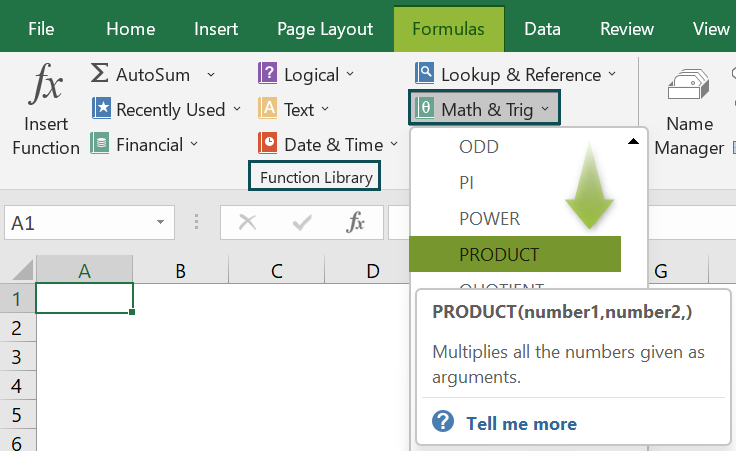2. How to apply the Excel PRODUCT function with conditions?

We can apply the Excel PRODUCT function with conditions by using it along with the IF().

Let us see the steps with an example.

The table below shows the order details of a set of laptops.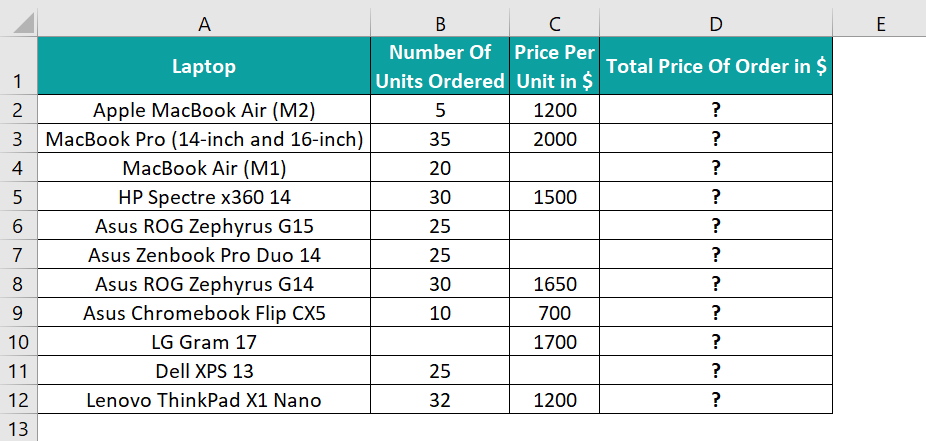And suppose the requirement is to determine the total order price for each laptop and display the output in column D. But, the conditions are that the number of orders placed for a laptop should be greater than 15. And the data entered for the specific laptop should be complete.

Then the steps to apply the PRODUCT() with the IF() in the target cells are as follows:

• Step 1: Select the target cell D2, enter the below formula, and press Enter.
=IF(B2>15,IF(OR(B2=””,C2=””),”Incomplete Data”,PRODUCT(B2,C2)),”NA”)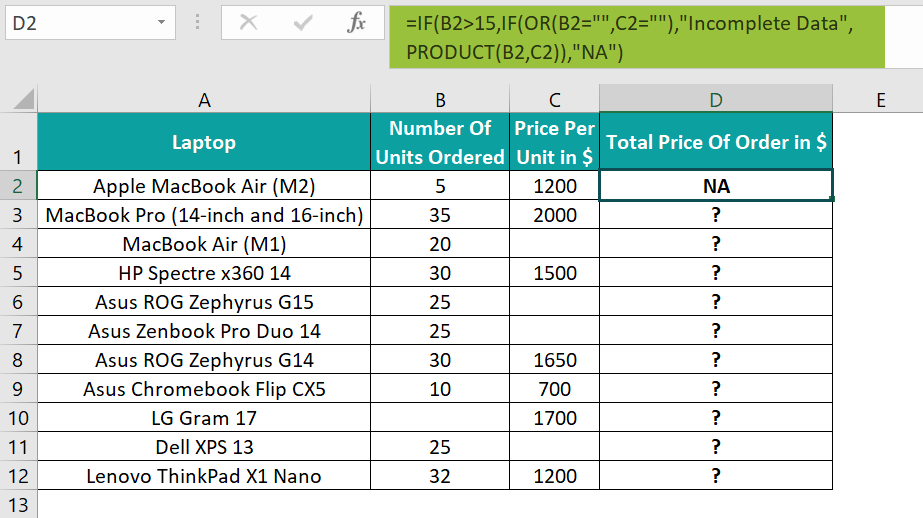• Step 2: Using the fill handle, copy the formula in the cell range D3:D12.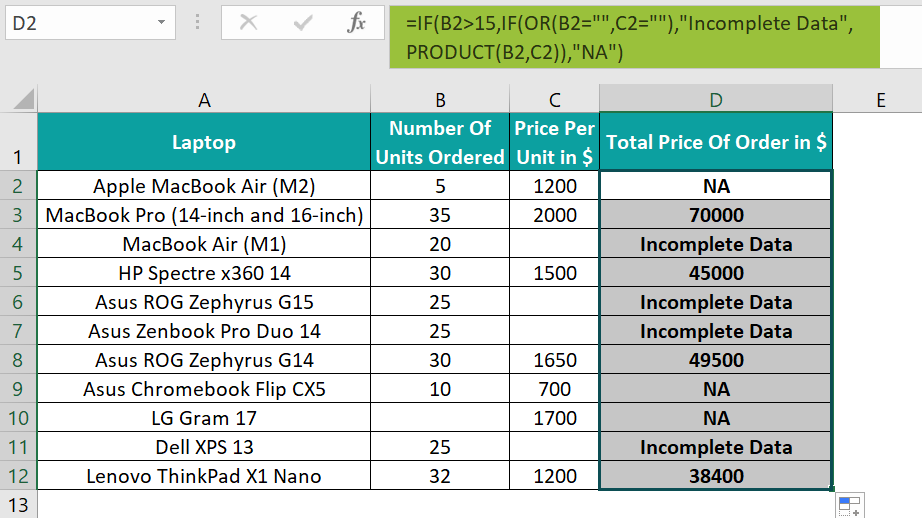In the above example, the outer IF() checks if the number of units ordered for the specific laptop is greater than 15. And if the condition holds, the inner IF() gets executed in the target cell.

Then the inner IF() checks if the column B or C cells in the specific row are blank. And if even one cell is blank, then the IF() returns the output as Incomplete Data. Otherwise, the PRODUCT() executes to return the product of the number of units ordered and the price per unit as the total price of the specific laptop order.

And if the outer IF() condition is false, the function returns the output as NA.

3. How can you apply the PRODUCT function in Excel VBA?

We can apply the PRODUCT function in Excel VBA using the method:

Application.WorksheetFunction.Product(number1,number2,..)

The arguments mentioned in the above method have the same interpretation as explained in the PRODUCT() Excel Formula section of the above article. However, we can enter up to 30 arguments.

4. What is the use of the PRODUCT function in Excel?

The use of the PRODUCT function in Excel is that it multiplies numbers in a wide cell range, even if the cell range contains empty cells or cells with non-numeric values.